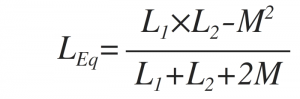Home > electronic components > inductors > inductors in parallel combination

# Inductors in Parallel Combination

Inductors can be connected in parallel combination when the terminals of individual inductors are connected to parallel to each terminal of the other inductors. Tin parallel combination of inductors the voltage drop across all the inductors is equal. Therefore, the inductors in parallel combination have equal voltage across the each inductor. Therefore, the voltage across the parallel combination of the inductors is given by,Figure below shows the parallel combination of inductors L1, L2, L3 and L4 ,As we know that in series combination of inductors the equivalent inductance is equal to the to the sum of all the individual inductors. On the other hand in the parallel combination of inductors the equivalent inductor is calculated differently. In parallel combination of inductors the current flowing through the each inductor is given by,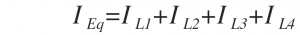As we know that, the self-induced emf across an inductor is given by,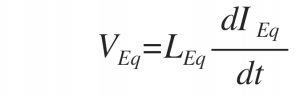Hence, by substituting the values of the individual currents flowing through each inductor in our circuit, voltage across the parallel combination is given by,By substituting the value of di/dt in from the relation between the inductance equation
i.e.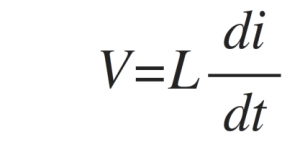We get,Therefore, in the parallel combination of the inductors the equivalent inductor is given by,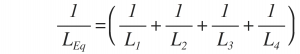If n number of inductors are connected in parallel, then the equivalent inductance of the parallel combination is given as,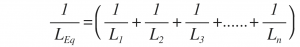Hence similar to parallel combination of resistors, the reciprocal value of the individual inductances is added together. However, in this parallel combination of inductors also there should be no mutual inductance or magnetic coupling between two or more inductors should be present. When there is coupling between the individual coils connected in parallel, the equivalent inductance will be affected because of the mutual coupling.
The above equation of calculating the equivalent inductance is normally used when more than two inductive coils are connected in parallel. If there are only two individual inductive coils are connected in parallel then the equivalent inductance can be calculated by the simple equation given below,Mutually Coupled Inductors in Parallel
In parallel combination of inductors the magnetic field of one inductor links with the other inductor this effect is known as mutual inductance effect. Because of this equivalent inductance of the parallel combination either increase or decrease depending upon the mutual coupling of the inductive coils. The impact of this mutual inductance depend on the distance between the coils and the orientation of the coils. Mutually connected inductors in parallel may aid or oppose the equivalent inductance of the parallel combination. Parallel aiding coils increase the equivalent inductance and parallel opposing coils decrease the equivalent inductance of the parallel combination of the individual inductors. The mutual coupled parallel inductive coils are shown either connected in an aid or oppose configuration is shown by the polarity dots. Figure below shows the parallel aiding and parallel opposing inductor combinations.As we know that, the voltage across the two parallel aiding inductors should be equal. Therefore, the equivalent inductance, for two parallel aiding inductors is given by,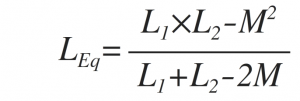Further, the equivalent inductance, for two parallel opposing inductors is given by,